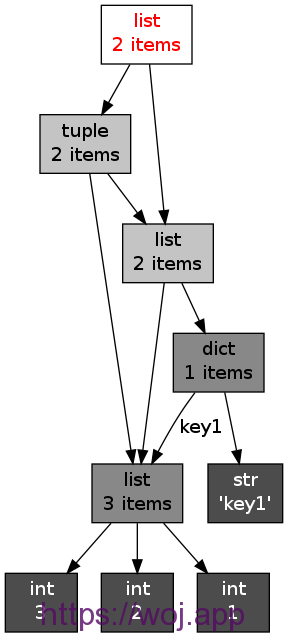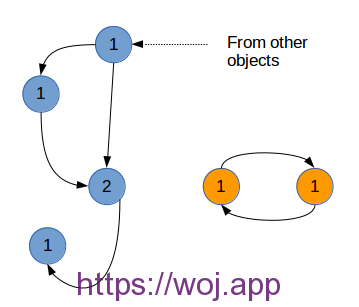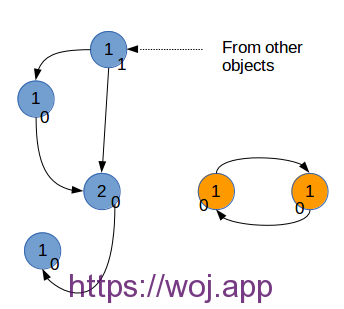A-A+

# Python 内存管理及删除or释放

2019年04月02日 22:02 阅读 187 views 次

### 对象的内存使用

`a = 1````a = 1

print(id(a))
print(hex(id(a)))```

11246696
'0xab9c68'

```a = 1
b = 1

print(id(a))
print(id(b))```

11246696

11246696

```# True
a = 1
b = 1
print(a is b)

# True
a = "good"
b = "good"
print(a is b)

# False
a = "very good morning"
b = "very good morning"
print(a is b)

# False
a = []
b = []
print(a is b)```

```from sys import getrefcount

a = [1, 2, 3]
print(getrefcount(a))

b = a
print(getrefcount(b))```

### 对象引用对象

Python的一个容器对象(container)，比如表、词典等，可以包含多个对象。实际上，容器对象中包含的并不是元素对象本身，是指向各个元素对象的引用。

```class from_obj(object):
def __init__(self, to_obj):
self.to_obj = to_obj

b = [1,2,3]
a = from_obj(b)
print(id(a.to_obj))
print(id(b))```

```from sys import getrefcount

a = [1, 2, 3]
print(getrefcount(a))

b = [a, a]
print(getrefcount(a))```

```x = [1, 2, 3]
y = [x, dict(key1=x)]
z = [y, (x, y)]

import objgraph
objgraph.show_refs([z], filename='ref_topo.png')```objgraph是Python的一个第三方包。安装之前需要安装xdot。

```sudo apt-get install xdot
sudo pip install objgraph```

objgraph官网

```a = []
b = [a]
a.append(b)```

```a = []
a.append(a)
print(getrefcount(a))```

### 引用减少

```from sys import getrefcount

a = [1, 2, 3]
b = a
print(getrefcount(b))

del a
print(getrefcount(b))```

del也可以用于删除容器元素中的元素，比如:

```a = [1,2,3]
del a
print(a)```

```from sys import getrefcount

a = [1, 2, 3]
b = a
print(getrefcount(b))

a = 1
print(getrefcount(b))```

### 垃圾回收

python话说会自己管理内存，实际上，对于占用很大内存的对象，并不会马上释放。举例，a=range(10000*10000)，会发现内存飙升一个多G，del a 或者a=[]都不能将内存降下来。。

del 可以删除多个变量，del a,b,c,d

import gc （garbage collector）
del a
gc.collect()

)。```a = [1, 2, 3]
del a```

del a后，已经没有任何引用指向之前建立的[1, 2, 3]这个表。用户不可能通过任何方式接触或者动用这个对象。这个对象如果继续待在内存里，就成了不健康的脂肪。当垃圾回收启动时，Python扫描到这个引用计数为0的对象，就将它所占据的内存清空。

```import gc
print(gc.get_threshold())```

### 分代回收

Python同时采用了分代(generation)回收的策略。这一策略的基本假设是，存活时间越久的对象，越不可能在后面的程序中变成垃圾。我们的程序往往会产生大量的对象，许多对象很快产生和消失，但也有一些对象长期被使用。出于信任和效率，对于这样一些“长寿”对象，我们相信它们的用处，所以减少在垃圾回收中扫描它们的频率。Python将所有的对象分为0，1，2三代。所有的新建对象都是0代对象。当某一代对象经历过垃圾回收，依然存活，那么它就被归入下一代对象。垃圾回收启动时，一定会扫描所有的0代对象。如果0代经过一定次数垃圾回收，那么就启动对0代和1代的扫描清理。当1代也经历了一定次数的垃圾回收后，那么会启动对0，1，2，即对所有对象进行扫描。

```import gc
gc.set_threshold(700, 10, 5)```

### 孤立的引用环

```a = []
b = [a]
a.append(b)

del a
del b```### 总结

Python作为一种动态类型的语言，其对象和引用分离。这与曾经的面向过程语言有很大的区别。为了有效的释放内存，Python内置了垃圾回收的支持。Python采取了一种相对简单的垃圾回收机制，即引用计数，并因此需要解决孤立引用环的问题。Python与其它语言既有共通性，又有特别的地方。对该内存管理机制的理解，是提高Python性能的重要一步。

### 布施恩德可便相知重

#### 微信扫一扫打赏#### 支付宝扫一扫打赏×